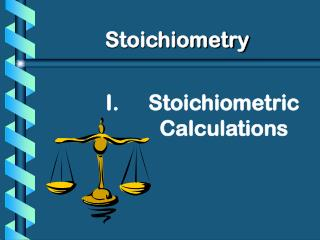Download PresentationStoichiometric Calculations# Stoichiometric Calculations - PowerPoint PPT Presentation

Download Presentation##### Stoichiometric Calculations

Download Policy: Content on the Website is provided to you AS IS for your information and personal use and may not be sold / licensed / shared on other websites without getting consent from its author. While downloading, if for some reason you are not able to download a presentation, the publisher may have deleted the file from their server.

- - - - - - - - - - - - - - - - - - - - - - - - - - - E N D - - - - - - - - - - - - - - - - - - - - - - - - - - -
##### Presentation Transcript

1. Stoichiometry Stoichiometric Calculations

2. A. Proportional Relationships Ratio of eggs to cookies • I have 5 eggs. How many cookies can I make? 2 1/4 c. flour 1 tsp. baking soda 1 tsp. salt 1 c. butter 3/4 c. sugar 3/4 c. brown sugar 1 tsp vanilla extract 2 eggs 2 c. chocolate chips Makes 5 dozen cookies. 5 eggs 5 doz. 2 eggs = 12.5 dozen cookies

3. A. Proportional Relationships • Stoichiometry • mass relationships between substances in a chemical reaction • based on the mole ratio • Mole Ratio • indicated by coefficients in a balanced equation 2 Mg + O2 2 MgO

4. B. Stoichiometry Steps 1. Write a balanced equation. 2. Identify known & unknown. 3. Line up conversion factors. • Mole ratio - moles  moles • Molar mass - moles  grams • Molarity - moles  liters soln • Molar volume - moles  liters gas • Mole ratio - moles  moles Core step in all stoichiometry problems!! 4. Check answer.

5. C. The Mole Hill Mole Ratio Molar Mass X Molar Mass Y Grams of X Grams of Y

6. C. Moles of X to Moles of Y • How many moles of H2O are produced from 10 moles of O2 gas? 2H2 + O2 2H2O 10 mol ? mol Mole Ratio 10 mol O2 2 mol H2O 1 mol O2 = 20 moles H2O

7. C. Moles of X to Grams of Y How many grams of NaOH are produced from 5 moles of Na? 2H2O + 2Na  2 NaOH + H2 ? grams 5 mol STEP 1 STEP 2 Formula Mass 2 mol NaOH 39.98 g NaOH 5 mol Na = 1 mol NaOH 2 mol Na 199.90 g NaOH Mole Ratio

8. C. Grams of X to Moles of Y How many moles of water are produced from 5 grams of oxygen gas? 4 NH3(g) + 5O2(g)  4NO(g) + 6H2O(g) ? moles 5 g STEP 2 STEP 1 5 g O2 1 mol O2 6 mol H2O 0.188 mol H2O = 5 mol O2 31.98 g O2 Mole Ratio Formula Mass

9. C. Grams of X to Grams of Y How many grams of MgO are produced from 145 g of Mg?2 Mg + O2 2 MgO ? g 145 g STEP 2 STEP 3 STEP 1 1 mol Mg 40.30 g MgO 2 mol MgO 145 g Mg 24.31g Mg 2 mol Mg 1 mol MgO Mole Ratio = 240.37 g MgO

10. LITERS OF GAS AT STP Molar Volume (22.4 L/mol) MASS IN GRAMS NUMBER OF PARTICLES MOLES Molar Mass (g/mol) 6.02  1023 particles/mol Molarity (mol/L) LITERS OF SOLUTION

11. Practice Problems

12. D. Stoichiometry Practice • How many moles of KClO3 must decompose in order to produce 9 moles of oxygen gas? 2KClO3 2KCl + 3O2 ? mol 9 mol 9 mol O2 2 mol KClO3 3 mol O2 = 6 mol KClO3

13. D. Stoichiometry Practice 3.How many grams of silver will be formed from 12.0 g copper? Cu + 2AgNO3 2Ag + Cu(NO3)2 12.0 g ? g 12.0 g Cu 1 mol Cu 63.55 g Cu 2 mol Ag 1 mol Cu 107.87 g Ag 1 mol Ag = 40.7 g Ag